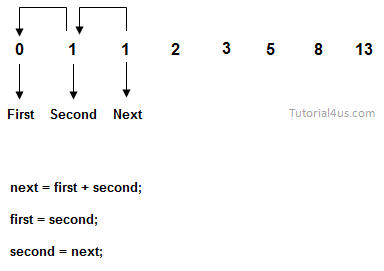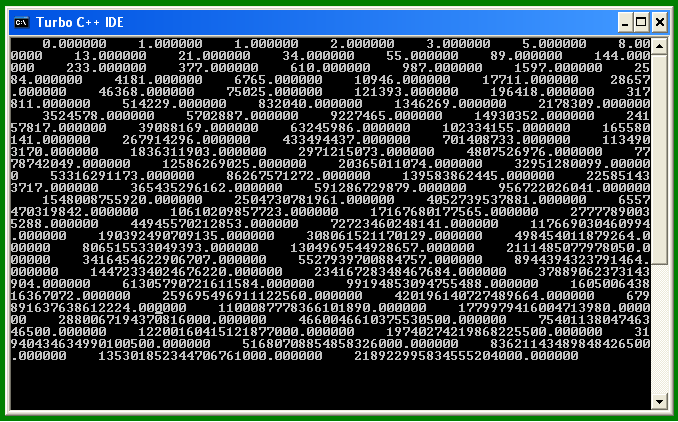# Fibonacci Series Program in C++

## C++ Program to Generate Fibonacci Series

Fibonacci Series is in the form of 0, 1, 1, 2, 3, 5, 8, 13, 21,...... To find this series we add two previous terms/digits and get next term/number.## Fibonacci Series Program in C++

```#include<iostream.h>
#include<conio.h>

void main()
{
int i,no, first=0, second=1, next;
clrscr();
first=0;
second=1;
cout<<"Enter nubmer of terms for Series: ";
cin>>no;
cout<<"Fibonacci series are: \n";
for(i=0; i<no; i++)
{
cout<<"\n"<<first;
next = first + second;
first = second;
second = next;
}
getch();
}
```

## Output

```Enter nubmer of terms for Series: 7
Fibonacci series are:
0
1
1
2
3
5
8
```

## Example

```#include<iostream.h>
#include<conio.h>

void main()
{
double i, first, second, next;
clrscr();
first=0;
second=1;
for(i=1;i<=100;i++)
{
cout<<first;
next=first+second;
first=second;
second=next;
}
getch();
}
```

Output## Pure VPN Privide Lowest Price VPN Just @ \$1.65. Per Month with Non Detected IP Lowest Price Non Detected IP VPN